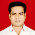Software Craftsman's Blog by Marcin Pieciukiewicz
Java and Scala development

## Monday, July 1, 2013

### List sorting in Scala

While I was watching Jacek Laskowski (polish blog) working on taskassin (also in polish) and Scala programming I decided to summarize different approches to List sorting in Scala.
In Jacek's problem we have to sort a list of tasks that are defined this way: (I simplified the class for the purpose of this article):

```case class Task(label: String, due: DateTime)
```
and we have defined list of objects:
```val tasks = List(Task("t1", DateTime.now),
Task("t2", DateTime.now + 2.hours),
Task("t3", DateTime.now - 2.hours))
```
Clarification: DateTime and .hours are from nscala-time library that encapsulates JodaTime so it can be easily used in Scala.

On a first glance we can see, that List class have three sorting methods:
.sorted - to sort objects with defined Ordered trait
.sortBy - to sort objects by selected field
.sortWith - to sort objects by given ordering function
Which one we will choose should depend on the business context of the application. Let's look at some situations, in which we might need to sort tasks list, that might occur:

Case 1. If sorting of tasks will always be done based on the value of due field, we can use object oriented programming and extend Task class with Ordered[Task] trait:
```case class Task(label: String, due: DateTime) extends Ordered[Task] {
def compare(other: Task): Int = this.due.compareTo(other.due)
}
```
That way, the sorting of tasks list could be done this way:
```val sortedTasks = tasks.sorted
```
It's hard to imagine something easier from the programmer's, that uses the Task class, point of view.

Case 2.1. The different approach have to be made if we'll assume that tasks can be sorted in multiple ways, ie. by due time, or by a label. In this case we can pass a parameter to sorted method, that is an instantiation of Ordering[Task] with definition of the sorting order:
``` val sortedTasks = tasks.sorted(new Ordering[Task] {
def compare(a: Task, b: Task): Int = a.due.compareTo(b.due)
})
```

also, you can use an easier way to create Ordering object:
```val sortedTasks = tasks.sorted(Ordering.fromLessThan[Task](_.due < _.due))
```
Case 2.2. Foregoing approach might seem not very elegant, but you can use it to create convenient way to sort tasks. To do so we can define ways of sorting in a companion object using Ordering[Task]:
```case class Task(label: String, due: DateTime)

//full syntax of defining anonymous function
val orderingByDue = Ordering.fromLessThan((a:Task, b:Task) => a.due < b.due)
//short syntax of defining anonymous function
val orderingByLabel = Ordering.fromLessThan[Task](_.label < _.label)
}
```
In this case you can sort tasks this way:
```val tasksSortedByDue = tasks.sorted(Task.orderingByDue)
```

Case 3. If, for some reason, we don't want to force programmer to use our way of sortnig, the sortBy method might be used. It takes, as a parameter, a field of the object that will be used to determine object sorting (by passing a getter function for that field):
```val sortedTasks = tasks.sortBy((t:Task) => t.due)
```
Or you can use shorter and more readable syntax:
```val sortedTasks = tasks.sortBy(_.due)
```
Additionally you can define more than one field that will be used for sorting (ie. sorting persons first by family name and, if those are the same, by the first name). You do that by passing a tuple of fields you are interested in:
```val sortedTasks = tasks.sortBy((x) => (x.label, x.due))
```
In this case tasks will be sorted by name and, if some of them have the same name, by the due time.

Case 4.1. If solution from Case 3. is not enought for you (ie. sorting is more complicated), you can use sortBy method, that requires a function that compares two objects:

```val sortedTasks = tasks.sortWith((a:Task, b:Task) => a.due < b.due)
```
Case 4.2. It is important to notice, that in this case (alike in case 2.2.) it is possible to define those functions as an elements of a companion object:
```object Task {
val compareByDue = (a:Task, b:Task) => a.due < b.due
}
```
And it can be used this way:
```val sortedTasks = tasks.sortWith(Task.compareByDue)
```

Sorting algorithms. While if was looking closely to the sorting in Scala, I've started to wonder about used sorting algoriths.

Sorting of sequences (including List) by usage of sorted, sortBy or sortWith methods is made by the sorting algorithm provided by JDK, in particular by java.util.Arrays.sort(...) function. Scala rewrites the sequence to Array and it is passed to the sort function, than it is sorted in place. Than based on the sorted array a new sequence is created (based on a given sequence type, ie. List).

Sorting method provided by JDK, used to sort array of objects, uses a modified mergesort algorithm, that guarantees sorting stability, and complexity O(n) = n log(n). Additionally, if input array is partially sorted, a performance of that algorithm is much greater.

It is important to notice that java.util.Arrays.sort(...) function, in case of primitives' array, uses an optimized quicksort algorithm (as opposed to objects' array, because in case of primitives, it doesn't have to be stable), but to use it in Scala you have to call it manually.

Summary. My personal favor approaches to list sorting is presented in Case 1. and Case 2.2. bacause these allow us to define a business context, so the programmer won't have to discover it over and over (of course that will have more sense in more compilcated cases, than presented in taskassin). Additionally these approaches allow us to define properties of Task class, no it's behavior.
I know that solutions presented by me don't exhaust the topic of sorting in Scala, but I think that those are the most usefull ones.

Next article: Named and Default parameters inconsistency in Scala

#### 1 comment:

1.Thanks for such nice documentation...